## Modular Programming In QBASIC

Subject: Computer Science

#### Overview

The process of breaking a large program into small manageable tasks and designed them independently is called modular programming. A module program consists of a main module and one or more sub modules or procedures.This note provides an information about modular program in QBASIC.

#### Modular ProgrammingThe process of breaking a large program into small manageable tasks and designed them independently is called modular programming. A module program consists of a main module and one or more sub modules or procedures. Each sub module has a unique name. Modular Programming is a approach in which the program is divided into separate independent units is called modules.

A modular program consist of main module and sub-module.

1. Main module : Modular Programming structure consists of many modules,the program entry point is a module,which is located at the top of the order modules.This top level module is called main module. The main module is the controlling section of a modular programming.
2. Sub- module : Sub- module is a program which is written under the main module. A modular program may have one or more than one sub- module. It is called sub- program. Qbasic is also known as modular programming language because it allows the user to divide program into manageable and functional modules or blocks with the help of sub procedure and function procedure.

Merits of Modular Programming• It reduces the program code.
• It is possible to use single module in different places.
• It makes debugging easier.
• It makes the maintenance of the program easier.
• It reduces the chance of changing the value of variable accidentally.

#### Procedures In Basic

There are two types of procedures in BASIC. They are as follows:

Sub Procedure : A sub procedure is a small manageable and functional part of a program that performs specific tasks and does not return any value to the calling module. A sub program is written with SUB....END SUB statements.

Some important features of sub procedure are as follows:

• It does not return any value.
• Sub procedure name can't be used as variable.
• Arguments can be passed to the sun program by reference or value.

Function Procedure : A function procedure is small manageable and functional part of a program that performs the specific tasks and returns a single value to the main program or calling module. A function is written with FUNCTION....... END FUNCTION statement.

Some important features of function procedure are as follows:

• It returns a value.
• Function procedure name has type declaration sign.
• Arguments can be passed to the sub-program by reference or by value.

#### Arguments

The constant or variables enclosed in the parentheses of procedure call statement and that are supplied to the procedure are known as arguments.The argument can be passed to a procedure either by reference or by value method.

Formal and Actual Parameter: Formal parameters are used to specify or declare the type of data to be passed to the procedures either by sub or function procedure.

#### Parameters

Variables in sub procedure declaration which accept data or variables passed to them from the calling module are known as parameters. It is also known as formal parameter.

Local and Global variable : A variable which is defined in a module ans is not accessible to any other modules is known as local variable. A variable in main module which can be accessed from any module or procedure of a program is known as global variable.

##### Things to remember
• The process of breaking a large program into small manageable tasks and designed them independently is called modular programming.
• A module program consists of a main module and one or more sub modules or procedures. Each sub module has a unique name.
• A modular program consist of main module and sub-module.
• The constant or variables enclosed in the parentheses of procedure call statement and that are supplied to the procedure are known as arguments.
• Variables in sub procedure declaration which accept data or variables passed to them from the calling module are known as parameters.
• It includes every relationship which established among the people.
• There can be more than one community in a society. Community smaller than society.
• It is a network of social relationships which cannot see or touched.
• common interests and common objectives are not necessary for society.
##### Videos for Modular Programming In QBASIC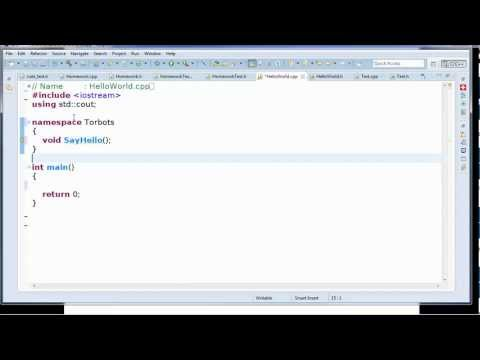##### C++ Lesson 02: Making Modular Code PT1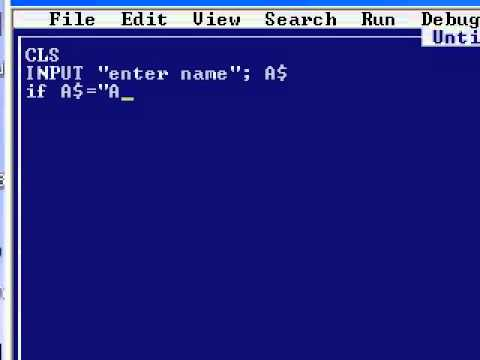##### How to make a simple qbasic program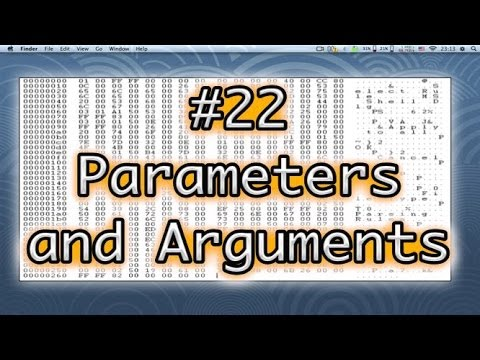##### Programming Basics #22 Parameters and Arguments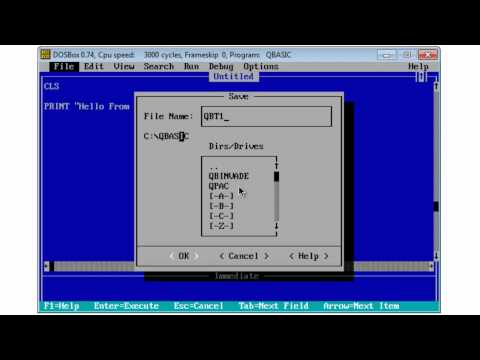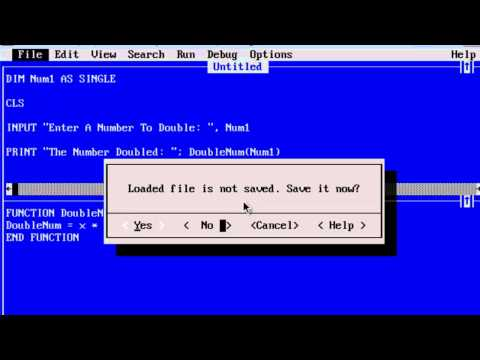##### QBasic Tutorial 38 - Making A Function - QB64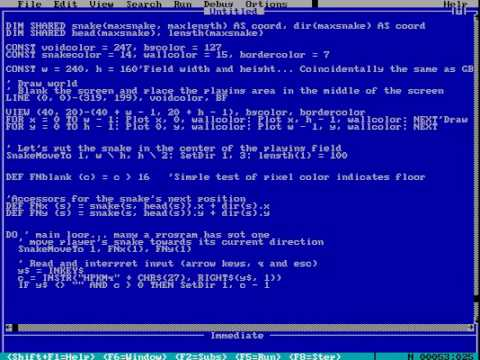##### Writing a QBasic game from scratch -- Snake game example
The differences between APPEND mode and OUTPUT mode are as follows:

 APPEND Mode OUTPUT Mode It is used to add more records in the existing sequential file. It is used to create new sequential data file and write data in it. It positions the file pointer to the end of the file. It positions the file pointer to the beginning of the file.
The differences between PRINT# and WRITE# is as follows:

 PRINT # WRITE # This statement is used to display each data. This statement is used to place the data into data file.
The modular programming is a programming technique in which a program is divided into many small logical, manageable and functional parts.
Any four advantages of modular programming are as follows:

• The debugging of the program becomes easier and faster.
• It improves readability of the program.
• The procedure can be tested and debugged separately.
• Individual procedure can be written independently so it is suitable for team work.
The sub module of a program is known as procedures. The types of procedures are as follows:

• SUB procedure
• FUNCTION procedure
The constants or variables enclosed in the parentheses of calling statement are known as arguments.
The variables enclosed in the parentheses of the procedure which accept constants or variables passed to them from the calling module are known as parameters.
Passing argument by reference method is the default method of passing arguments to the procedure. The addresses of the variables are passed to the procedure in this method. That means parameters use the same memory addresses of the arguments.
DECLARE SUB sum (A, B, C)
CLS
Input"enter the first number"; A
Input"enter the second number"; B
Input"enter the third number"; C
CALL sum
END
SUB sum (A, B, C)
S= A+B+C
Print"The sum of three number is"; S
END SUB
DECLARE SUB Temperature (F)
CLS
Input"Enter the temperature in Fahrenheit"; F
CALL Temperature (F)
END
SUB Temperature (F)
Temperature in Celsius= (5(f-32)/9)
Print"The temperature in Celsius"; Temperature in Celsius
END SUB
DECLARE SUB Interest (P, T, R)
CLS
Input"Enter the principle"; P
Input"Enter the time"; T
Input"Enter the rate"; R
CALL Interest (P, T, R)
END
SUB Interest (P, T, R)
I= (P*T*R)/100
Print"The simple interest is"; I
END SUB
DECLARE SUB Area (L, B)
CLS
Input"Enter the length"; L
CALL Area (L, B)
END
SUB Area (L, B)
A = (2*(L+B))
Print"The area of rectangle is"; A
END SUB
DECLARE SUB Area (R)
CLS
CALL Area (R)
END
SUB Area (R)
A= (4*3.14*R^2)
Print"The area of sphere is"; A
END SUB
DECLARE SUB GREATEST (NUM ())
CLS
DIM NUM (p)
Print"The greatest number is:"
For p= 1 to 3
Input NUM (p)
Next p
Call GREATEST (NUM ())
END
DECLARE FUNCTION Longest$(name$ ())
CLS
DIM name$(3) For p= 1 to 3 Input"Enter the name"; name$ (p)
Next p
Print"The longest name is"; Longest$(name$ ())
END
FUNCTION Longest$(name$ ())
Let m$= name$ (1)
For p = 2 to 3
IF LEN (name$(p))> LEN (m$) THEN
m $= name$ (p)
END IF
Next p
Longest$= m$
END FUNCTION
DECLARE SUB Rev(A$) CLS INPUT"Enter any string";A$
CALL Rev(A$) END SUB Rev(A$)
END
SUB Rev(A$) FOR I = LEN(A$) to 1 Step-1
B$=B$ + MID$(A$,I,1)
NEXT I
PRINT"Reverse string is";B$END SUB DECLARE SUB Pal (A$)
CLS
Input"Enter any string"; A$CALL Pal (A$)
END
SUB Pal (A$) For I= LEN (A$) TO 1 step-1
B$= B$+MID$(A$, I, 1)
Next I
IF A$= B$ THEN
Print"It is palindrome"
ELSE
Print"IT is not palindrome"
END IF
END SUB

DECLARE FUNCTION Vowel$(A$)
CLS
INPUT"Enter any string";A$PRINT Vowel(A$)
END
FUNCTION Vowel$(A$)
FOR I = 1 to LEN(A$) B$ = U case$(MID$(A$,I,1) IF B$<>"A" AND B$<>"E" AND B$ <> "I" AND B$<> "O" AND B$ <> "U" THEN C$=C$+B$NEXT I Vowel$= C$ENDIF END FUNCTION DECLARE SUB KINS (A$)
CLS
A$="KATHMANDU" CALL KINS (A$)
END
SUB KINS (A$) T=9 P=1 FOR I= 5 TO 1 STEP -1 PRINT TAB (T); MID$ (A$, I, P) T= T-1 P=P+2 NEXT I END SUB DECLARE SUB KINS (A$)
CLS
A$="KATHMANDU" CALL KINS (A$)
END
SUB KINS (A$) T=1 P=9 FOR I= 1 TO 5 PRINT TAB (T); MID$ (A$, T, P) T=T+1 P=P-2 NEXT I END SUB DECLARE SUB KINS (A$)
CLS
A$="WELCOME" CALL KINS (A$)
END
SUB KINS (A$) FOR I= 1 to LEN (A$)
PRINT Right$(A$, 1)
NEXT I
END SUB
DECLARE SUB KINS (A$) CLS A$="WELCOME"
CALL KINS (A$) END SUB KINS (A$)
FOR I= 1 to LEN (A$) PRINT left$ (A$, 1) NEXT I END SUB DECLARE FUNCTION AREA (L, B) CLS Input"Enter the length"; L Input"Enter the breadth"; B Print"The area is"; AREA (L, B) END FUNCTION AREA (L, B) AREA= L*B END FUNCTION DECLARE FUNCTION AOC (R) CLS Input"Enter the radius"; R Print"The area is"; AOC (R) END FUNCTION AOC (R) CONST PI= 22/7 AOC= PI*R^2 END FUNCTION DECLARE FUNCTION TEST$ (n)
CLS
Input"Enter a number"; n
Result$=TEST$ (n)
Print n;"is a"; result $END FUNCTION TEST$ (n)
IF n>0 THEN
R$="positive" ELSEIF n<0 THEN R$="zero"
END IF
TEST$= R$
END FUNCTION
DECLARE FUNCTION TSA ( R,H )
DECLARE FUNCTION V (R,H )
CLS
INPUT"Enter the height";H
PRINT TSA (R,H)
Print V ( R,H )
END
FUNCTION TSA ( R,H )
T= 2* 22/7* R* ( R + H )
TSA= T
END FUNCTION
FUNCTION V ( R,H )
Vol= 22/7*R^2*H
V = Vol
END FUNCTION

DECLARE SUB Area ( l )
CLS
INPUT"Enter the length";l
CALL Area ( l )
END
SUB Area ( l )
A = l^ 2
PRINT" Area of square"; A
END SUB

REM fibonacci series 0,1,1,2,3,5,....,10th terms DECLARE SUB fibo(m,n) CLS a = 0 b = 1 PRINT a; b; CALL fibo(a, b) END SUB fibo (a, b) FOR i = 1 TO 8 c = a + b PRINT c; SWAP a, b SWAP b, c NEXT i END SUB